# Cost Volume Profit CVP Analysis

## Cost Volume Profit CVP Analysis

### Cost Volume Profit CVP Analysis

Chapter 7 Cost Volume Profit (CVP) Analysis

1.      Objectives

1.1       Explain the nature of CVP analysis.
1.2       Calculate and interpret breakeven point and margin of safety.
1.3       Calculate the contribution to sales ratio, in single and multi-product situations, and demonstrate an understanding of its use.
1.4       Calculate target profit or revenue in single and multi-product situations, and demonstrate an understanding of its use.
1.5       Prepare break even charts and profit volume charts and interpret the information contained within each, including multi-product situations
1.6       Discuss the limitations of CVP analysis for planning and decision making.2.      A Recap of Basic CVP Analysis for Single Product

2.1       Breakeven analysis

2.1.1

Key Terms

(a)       Contribution per unit = unit selling price – unit variable costs

(b)       Breakeven point = activity level at which there is neither profit nor loss
=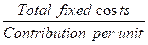(c)       Contribution/sales (C/S) ratio = profit/volume (P/V) ratio
=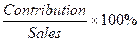(d)       Sales revenue at breakeven point = fixed costs ÷ C/S ratio

(e)       Margin of safety (in units) = budgeted sales units – breakeven sales units

 (f) Margin of safety (as %) Budgeted sales – breakeven sales × 100% Budgeted sales
 (g) Sales volume to achieve a target profit Fixed cost + target profit Contribution per unit

The Graphical Approach for Single Product

2.2.1    The second way to find the break-even is to use the graphical method. The graphical method is based on the break-even chart, a graphical representation of cost-volume-profit relationships and the break-even point. It is an attempt to help management in their understanding of these relationships and so enable them to decide on the optimum level of output.

2.2.2

# Example 1

A new product has the following sales and cost data.

 Selling price \$60 per unit Variable costs \$40 per unit Fixed costs \$25,000 per month

Forecast sales 1,800 units per month

Required:
Prepare a breakeven chart using the above data.

Solution: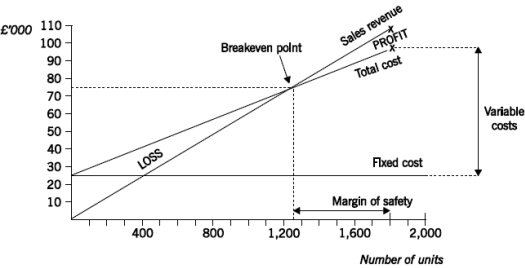3.      Breakeven Analysis for Multiple Products

3.1       A major assumption

3.1.1    Organisations typically produce and sell a variety of products and services. To perform breakeven analysis in a multi-product organisation, however, a constant product sales mix must be assumed. In other words, we have to assume that whenever x units of product A are sold, y units of product B and z units of product C are also sold.

3.1.2

# Example 2

PL produces and sells two products. The M sells for \$7 per unit and has a total variable cost of \$2.94 per unit, while the N sells for \$15 per unit and has a total variable cost of \$4.5 per unit. The marketing department has estimated that for every five units of M sold, one unit of N will be sold. The organization’s fixed costs total \$36,000.

Required:

Calculate the breakeven point for PL.

Solution:
1.         Calculate contribution per unit

 M N \$ per unit \$ per unit Selling price 7.00 15.00 Variable cost 2.94 4.50 Contribution 4.06 10.50

2.         Calculate contribution per mix
= \$4.06 x 5 + \$10.50 x 1 = \$30.80

3.         Calculate the breakeven point in terms of the number of mixes
= fixed costs / contribution per mix
= \$36,000 / \$30.80
= 1,169 mixes (rounded)

4.         Calculate the breakeven point in terms of the number of units of products
= (1,169 x 5) 5,845 units of M and (1,169 x 1) 1,169 units of N

5.         Calculate the breakeven point in terms of revenue
= (5,845 x \$7) + (1,169 x \$15)
= \$40,915 of M and \$17,535 of N
= \$58,450 in total

3.1.3

# Exercise 2

Alpha manufactures and sells three products, the beta, the gamma and the delta. Relevant information is as follows.

 Beta Gamma Delta \$ per unit \$ per unit \$ per unit Selling price 135.00 165.00 220.00 Variable cost 73.50 58.90 146.20

Total fixed costs are \$950,000.

An analysis of past trading patterns indicates that the products are sold in the ratio 3:4:5

Required:

Calculate the breakeven point for Alpha.

3.2       Contribution to sales (C/S) ratio for multiple products

3.2.1    The breakeven point in terms of sales revenue can be calculated as fixed costs / average C/S ratio.
3.2.2    Any change in the proportions of products in the mix will change the contribution per mix and the average C/S ratio and hence the breakeven point.
3.2.3    You should know that the C/S ratio is sometimes called the profit/volume ratio or P/V ratio.

3.2.4

# Example 3

As example 2 above, we can calculate the breakeven point of PL as follows.

Solution:
1.         Calculate revenue per mix
= (5 x \$7) + (1 x \$15) = \$50

2.         Calculate contribution per mix (see example 2)
= \$4.06 x 5 + \$10.50 x 1 = \$30.80

3.         Calculate average C/S ratio
= \$30.80/\$50.00 x 100%
= 61.6%

4.         Calculate the breakeven point
= fixed costs ÷ C/S ratio
= \$36,000 ÷ 0.616
= \$58,443 (rounded)

5.         Calculate revenue ratio of mix
= (5 x \$7) : (1 x \$15)
= 35 : 15 or 7 : 3

6.         Calculate breakeven sales
M = \$58,442 x 7/10 = \$40,909 (rounded)
N = \$58,442 x 3/10 = \$17,533 (rounded)

3.2.5

# Exercise 3

Calculate the breakeven sales revenue of product Beta, Gamma and Delta (see Exercise 2 above) using the approach shown in Example 3.

 Solution:

 3.2.6 Points to Bear in Mind Any change in the proportions of products in the mix will change the contribution per mix and the average C/S ratio and hence the breakeven point. (a)       If the mix shifts towards products with lower contribution margins, the breakeven point (in units) will increase and profits will fall unless there is a corresponding increase in total revenue. (b)       A shift towards products with higher contribution margins without a corresponding decrease in revenues will cause an increase in profits and a lower breakeven point. (c)       If sales are at the specified level but not in the specified mix, there will be either a profit or a loss depending on whether the mix shifts towards products with higher or lower contribution margins.

3.3       Margin of safety for multiple products

3.3.1    The margin of safety for a multi-product organisation is equal to the budgeted sales in the standard mix less the breakeven sales in the standard mix. It may be expressed as a percentage of the budgeted sales.

3.3.2

# Example 4

BA produces and sells two products. The W sells for \$8 per unit and has a total variable cost of \$3.80 per unit, while the R sells for \$14 per unit and has a total variable cost of \$4.20. For every five units of W sold, six units of R are sold. BA's fixed costs are \$43,890 per period.

Budgeted sales revenue for next period is \$74,400, in the standard mix.

Required:

Calculate the margin of safety in terms of sales revenue and also as a percentage of budgeted sales revenues.

Solution:

1.         Calculate contribution per unit

 W R \$ per unit \$ per unit Selling price 8.00 14.00 Variable cost 3.80 4.20 Contribution 4.20 9.80

2.         Calculate contribution per mix
= \$4.20 x 5 + \$9.80 x 6 = \$79.80

3.         Calculate the breakeven point in terms of the number of mixes
= fixed costs / contribution per mix
= \$43,890 / \$79.80
= 550 mixes

4.         Calculate the breakeven point in terms of the number of units of products
= (550 x 5) 2,750 units of W and (550 x 6) 3,300 units of R

5.         Calculate the breakeven point in terms of revenue
= (2,750 x \$8) + (3,300 x \$14)
= \$22,000 of W and \$46,200 of R
= \$68,200 in total

6.         Calculate the margin of safety
= budgeted sales – breakeven sales
= \$74,400 – \$68,200
= \$6,200 sales in total, in the standard mix
= \$6,200 / \$74,400 x 100%
= 8.3% of budgeted sales

3.4       Target profits for multiple products
3.4.1    The number of mixes of products required to be sold to achieve a target profit is calculated as:

 (fixed costs + required profit)/contribution per mix.

3.4.2

# Example 5

An organisation makes and sells three products, F, G and H. The products are sold in the proportions F:G:H = 2:1:3. The organisation's fixed costs are \$80,000 per month and details of the products are as follows.

 Selling price Variable cost Product \$ per unit \$ per unit F 22 16 G 15 19 H 19 13

The organisation wishes to earn a profit of \$52,000 next month. Calculate the required sales value of each product in order to achieve this target profit.

Solution:
1.         Calculate contribution per unit

 F G H \$ per unit \$ per unit \$ per unit Selling price 22 15 19 Variable cost 16 12 13 Contribution 6 3 6

2.         Calculate contribution per mix
= (\$6 x 2) + (\$3 x 1) + (\$6 x 3)
= \$33

3.         Calculate the required number of mixes
= (Fixed costs + required profit)/contribution per mix
= (\$80,000 + \$52,000)/\$33
= 4,000 mixes

4.         Calculate the required sales in terms of the number of units of the products and sales revenue of each product

 Product Units Selling price Sales revenue required \$ per unit \$ F 4,000 x 2 8,000 22 176,000 G 4,000 x 1 4,000 15 60,000 H 4,000 x 3 12,000 19 228,000 Total 464,000

The sales revenue of \$464,000 will generate a profit of \$52,000 if the products are sold in the mix 2:1:3.

Alternatively the C/S ratio could be used to determine the required sales revenue for a profit of \$52,000. The method is again similar to that demonstrated earlier when calculating the breakeven point.

3.4.3

# Example 6

Using the information as Example 5, calculate the required sales of each products by using the C/S ratio.

Solution:
1.         Calculate revenue per mix
= (2 x \$22) + (1 x \$15) + (3 x \$19)
= \$116
2.         Calculate contribution per mix
= (\$6 x 2) + (\$3 x 1) + (\$6 x 3)
= \$33
3.         Calculate average C/S ratio
= (\$33/\$116) x 100%
= 28.45%
4.         Calculate the required total revenue
= required contribution ÷ C/S ratio
= (\$80,000 + \$52,000) ÷ 28.45%
= \$463,972
5.         Calculate revenue ratio of mix
= (2 x \$22) : (1 x \$15) : (3 x \$19)
= 44 : 15 : 57
6.         Calculate required sales
Required sales of F = 44/116 x \$463,972 = \$175,989
Required sales of G = 15/116 x \$463,972 = \$59,996
Required sales of H = 57/116 x \$463,972 = \$227,986

Which, allowing for roundings, is the same answer as calculated in the first example.

3.5       Multi-product breakeven charts

(A)      Breakeven charts

3.5.1    Breakeven charts for multiple products can be drawn if a constant product sales mix is assumed.
3.5.2    Here, there are three approaches to draw the multi-product breakeven charts.

3.5.3

# Example 7 – Approach 1: Output in \$ Sales and a Constant Product Mix

Assume that budgeted sales are 2,000 units of X, 4,000 units of Y and 3,000 units of Z. A breakeven chart would make the assumption that output and sales of X, Y and Z are in the proportions 2,000: 4,000: 3,000 at all levels of activity, in other words that the sales mix is 'fixed' in these proportions.

We begin by carrying out some calculations.

 Budgeted costs Costs Revenue \$ \$ Variable costs of X (2,000 x \$3) 6,000 X (2,000 x \$8) 16,000 Variable costs of Y (4,000 x \$4) 16,000 Y (4,000 x \$6) 24,000 Variable costs of Z (3,000 x \$5) 15,000 Z (3,000 x \$6) 18,000 Total variable costs 37,000 Budgeted revenue 58,000 Fixed costs 10,000 Total budgeted costs 47,000

The breakeven chart can now be drawn.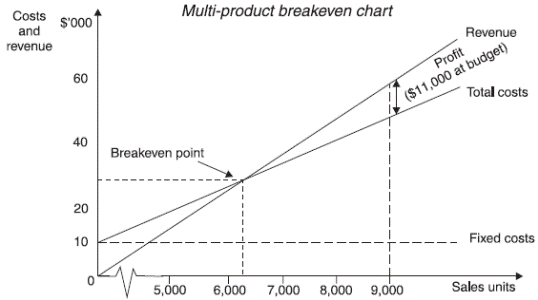The breakeven point is approximately \$27,500 of sales revenue. This may either be read from the chart or computed mathematically.
(a)       The budgeted C/S ratio for all three products together is contribution/sales = \$(58,000 – 37,000)/\$58,000 = 36.21%.
(b)       The required contribution to break even is \$10,000, the amount of fixed costs. The breakeven point is \$10,000/36.21% = \$27,500 (approx) in sales revenue.

The margin of safety is approximately \$(58,000 – 27,500) = \$30,500.

3.5.4

# Example 8 – Approach 2: Products in Sequence

The products could be plotted in a particular sequence (say X first, then Y, then Z). Using the data from Approach 1, we can calculate cumulative costs and revenues as follows.

 Product Cumulative units Cumulative costs Cumulative revenue \$ \$ Nil 10,000 Nil X (2,000 units) 2,000 16,000 16,000 Y (4,000 units) 6,000 32,000 40,000 Z (3,000 units) 9,000 47,000 58,000

The breakeven chart can now be drawn.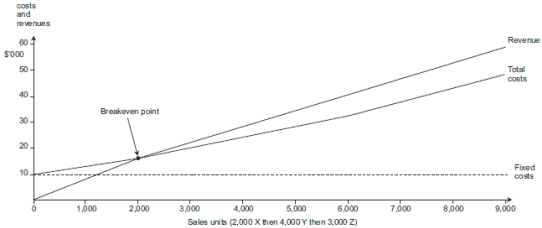In this case the breakeven point occurs at 2,000 units of sales (2,000 units of product X). The margin of safety is roughly 4,000 units of Y and 3,000 units of Z.

3.5.5

# Example 9 – Approach 3: Output in Terms of % of Forecast Sales and a Constant Product Mix

The breakeven point can be read from the graph as approximately 48% of forecast sales (\$30,000 of revenue).

Alternatively, with contribution of \$(58,000 – 37,000) = \$21,000, one percent of forecast sales is associated with \$21,000/100 = \$210 contribution.

Breakeven point (%) = fixed costs/contribution per 1%
= \$10,000/\$210 = 47.62%
∴Margin of safety = (100 – 47.62) = 52.38%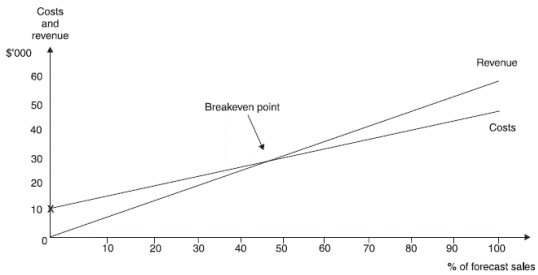(B)       Multi-product P/V charts

3.5.6    The same information could be shown on a P/V chart.

3.5.8

# Exercise 4

A company sells three products, X, Y and Z. Cost and sales data for one period are as follows.

 X Y Z Sales volume 2,000 units 2,000 units 5,000 units Sales price per unit \$3 \$4 \$2 Variable cost per unit \$2.25 \$3.50 \$1.25 Total fixed costs \$3,250

Required:

Construct a multi-product P/V chart based on the above information on the axes below.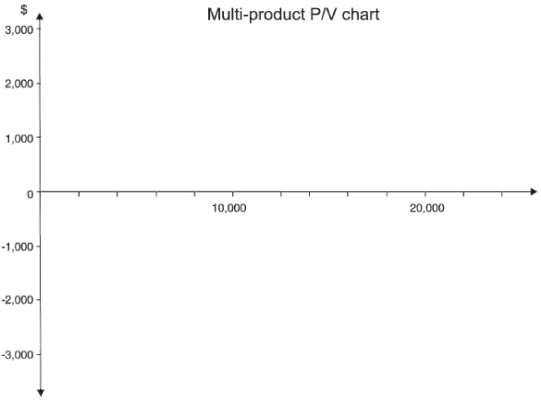4.       Further Aspects of CVP Analysis

4.1       The usefulness of CVP analysis is restricted by its unrealistic assumptions, such as constant sales price at all levels of activity. However CVP has the advantage of being more easily understood by non-financial managers due to its graphical depiction of cost and revenue data.

4.2       Limitations:
(a)        It is assumed that fixed costs are the same in total and variable costs are the same per unit at all levels of output. This assumption is a great simplification.
(i)        Fixed costs will change if output falls or increases substantially (most fixed costs are step costs).
(ii)       The variable cost per unit will decrease where economies of scale are made at higher output volumes, but the variable cost per unit will also eventually rise when diseconomies of scale begin to appear at even higher volumes of output (for example the extra cost of labour in overtime working).

(b)        The assumption is only correct within a normal range or relevant range of output. It is generally assumed that both the budgeted output and the breakeven point lie within this relevant range.
(c)        It is assumed that sales prices will be constant at all levels of activity. This may not be true, especially at higher volumes of output, where the price may have to be reduced to win the extra sales.
(d)       Production and sales are assumed to be the same, so that the consequences of any increase in inventory levels or of 'de-stocking' are ignored.
(e)        Uncertainty in the estimates of fixed costs and unit variable costs is often ignored.
(a)        Graphical representation of cost and revenue data (breakeven charts) can be more easily understood by non-financial managers.
(b)       A breakeven model enables profit or loss at any level of activity within the range for which the model is valid to be determined, and the C/S ratio can indicate the relative profitability of different products.
(c)        Highlighting the breakeven point and the margin of safety gives managers some indication of the level of risk involved.

Examination Style Questions

Question 1 – Breakeven Chart with Increases in Fixed Costs
(a)     Identify and discuss briefly five assumptions underlying cost-volume-profit analysis.
(10 marks)
(b)     A local authority, whose area include a holiday resort situated on the east coast, operates, for 30 weeks each year, a holiday home which is let to visiting parties of children in care from other authorities. The children are accompanied by their own house mothers who supervise them throughout their holiday. From six to fifteen guests are accepted on terms of £100 per person per week. No differential charges exist for adults and children.

Weekly costs incurred by the host authority are:

 £ per guest Food 25 Electricity for heating and cooking 3 Domestic (laundry, cleaning, etc.) expenses 5 Use of minibus 10

Seasonal staff supervise and carry out the necessary duties at the home at a cost of £11,000 for the 30-week period. This provides staffing sufficient for six to ten guests per week but if eleven or more guests are to be accommodated, additional staff at a total cost of £200 per week are engaged for the whole of the 30-week period.

Rent, including rates for the property, is £4,000 per annum and the garden of the home is maintained by the council’s recreation department which charges a nominal fee of £1,000 per annum.

Required:

(i)      Tabulate the appropriate figures in such a way as to show the break-even point(s) and to comment on your figures.                                                                                            (8 marks)
(ii)     Draw, on the graph paper provided, a chart to illustrate your answer to (b)(i) above. (7 marks)
(Total 25 marks)

Question 2 – Multi-product Profit-volume Graph
JK Limited has prepared a budget for the next 12 months when it intends to make and sell four products, details of which are shown below:

 Product Sales in units Selling price per unit Variable cost per unit (000) £ £ J 10 20 14.00 K 10 40 8.00 L 50 4 4.20 M 20 10 7.00

Budgeted fixed costs are £240,000 per annum and total assets employed are £570,000.

Required:

(a)     Calculate the total contribution earned by each product and their combined total contributions.     (2 marks)
(b)     Plot the data of your answer to (a) above in the form of a contribution to sales graph (or P/V graph) on the graph paper provided.                                                                                      (6 marks)
(c)     Explain your graph to management, to comment on the results shown and to state the break-even point.                                                                                                                               (4 marks)
(d)     Describe briefly three ways in which the overall contribution to sales ratio could be improved.     (3 marks)
(Total 15 marks)

Question 3
You are the assistant management accountant of QXY plc, a food manufacturer. The Board of Directors is concerned that its operational managers may not be fully aware of the importance of understanding the costs incurred by the business and the effect that this has on their operational decision making. In addition, the operational managers need to be aware of the implications of their pricing policy when trying to increase the volume of sales.

You are scheduled to make a presentation to the operational managers tomorrow to explain to them the different costs that are incurred by the business, the results of some research that has been conducted into the implications for pricing and the importance of understanding these issues for their decision making. The diagram on the next page has already been prepared for the presentation.

Required:

You are required to interpret the diagram and explain how it illustrates issues that the operational managers should consider when making decisions. (Note: your answer must include explanations of the Sales Revenue, Total Cost and Fixed Cost lines, and the significance of each of the activity levels labelled A, B, C, D.) (10 marks)

Question 4
RDF Ltd offers four services to television companies The number of services provided is measured in service units and details of RDF Ltd's draft budget for its year ending 30 June 2005 are as follows.

 Service K Service L Service M Service N No. of service units 1,000 2,300 1,450 1,970 Selling price per unit (\$) 18 16 12 20 Variable cost per unit (\$) 8 10 13 13 Fixed cost per unit (\$) 2 3 2 4

The budgeted level of activity shown in the table above has been based on fully meeting the forecasted market demand for each type of service.

The following chart has been prepared based on the draft budget above.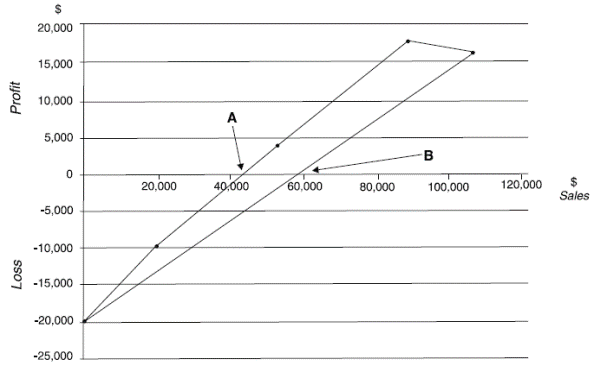Required:

(a)     Explain the meaning of the values shown as points A and B on the chart. (Note. Calculations are not required.)                                                                                                               (4 marks)
(b)     Further investigation into the nature of the fixed costs has shown that some of those shown in the original budget are incurred as a direct result of providing specific services as follows.

 \$ Service K 4,400 Service L 3,700 Service M Nil Service N 2,650

The remaining budgeted fixed costs are general fixed costs that will be incurred regardless of the type and number of services provided.

RDF Ltd entered into a three-year contract in June 2002 which requires it to provide 500 units of service M per year or suffer significant financial penalties. These services are included in the budgeted demand.

Required:

(i)      Evaluate the financial viability of each of the four services currently provided.
(6 marks)
(ii)     Recommend the operating plan that will maximise profit for the year ended 30 June 2005 and state the resulting profit. Explain the assumptions that led to your decision and other factors that should be considered.                                                                                              (5 marks)
(iii)    Calculate the overall breakeven sales value for the operating plan you have recommended in answer to (b)(ii), stating clearly the assumptions made in your calculations.         (5 marks)
(iv)    Comment on any limitations of using breakeven analysis for decision making purposes.      (5 marks)
(Total = 25 marks)

3.5.7

# Example 10

Same information as Example 7,

 Product Contribution Sales C/S ratio \$ \$ % X 10,000 16,000 62.50 Y 8,000 24,000 33.33 Z 3,000 18,000 16.67 Total 21,000 58,000 36.21

By convention, the products are shown individually on a P/V chart from left to right, in order of the size of their C/S ratio. In this example, product X will be plotted first, then product Y and finally product Z. A dotted line is used to show the cumulative profit/loss and the cumulative sales as each product's sales and contribution in turn are added to the sales mix.

 Product Cumulative sales Cumulative profit \$ \$ X 16,000 (\$16,000 – \$16,000) - X and Y 40,000 8,000 X, Y and Z 58,000 11,000

You will see on the graph which follows that these three pairs of data are used to plot the dotted line, to indicate the contribution from each product. The solid line which joins the two ends of this dotted line indicates the average profit which will be earned from sales of the three products in this mix.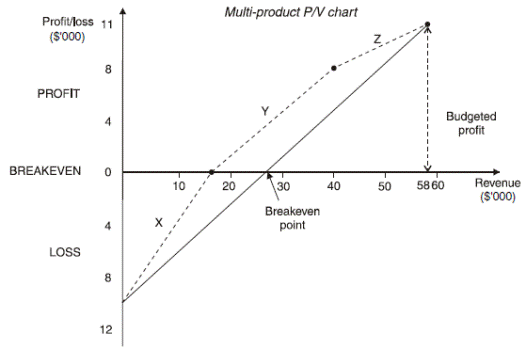The diagram highlights the following points.
(a)       Since X is the most profitable in terms of C/S ratio, it might be worth considering an increase in the sales of X, even if there is a consequent fall in the sales of Z.
(b)       Alternatively, the pricing structure of the products should be reviewed and a decision made as to whether the price of product Z should be raised so as to increase its C/S ratio (although an increase is likely to result in some fall in sales volume).

The multi-product P/V chart therefore helps to identify the following.
(a)       The overall company breakeven point.
(b)       Which products should be expanded in output and which, if any, should be discontinued.
(c)       What effect changes in selling price and sales volume will have on the company's breakeven point and profit.

2.1.2

# Exercise 1

A company manufactures a single product which has the following cost structure based on a production budget of 10,000 units.

 Materials – 4 kg at \$3/kg \$12 Direct labour – 5 hours at \$7/hour \$35

Variable production overheads are recovered at the rate of \$8 per direct labour hour.

Other costs incurred by the company are:

The selling and distribution overheads include a variable element due to a distribution cost of \$2 per unit.

The fixed selling price of the unit is \$129.

Required:
(a)    Calculate how many units have to be sold for the company to breakeven.
(b)    Calculate the sales revenue which would give a net profit of \$40,000.
(c)    If the company could buy in the units instead of manufacturing them, calculate how much it would be prepared to pay if both:
(i)     estimated sales for next year are 9,500 units at \$129 each; and
(ii)    \$197,500 of fixed selling, distribution and administrative overheads would still be incurred even if there is no production (all other fixed overheads would be saved).

Source: https://hkiaatevening.yolasite.com/resources/PMNotes/Ch7-CVP.doc

Web site to visit: https://hkiaatevening.yolasite.com

Author of the text: indicated on the source document of the above text

If you are the author of the text above and you not agree to share your knowledge for teaching, research, scholarship (for fair use as indicated in the United States copyrigh low) please send us an e-mail and we will remove your text quickly. Fair use is a limitation and exception to the exclusive right granted by copyright law to the author of a creative work. In United States copyright law, fair use is a doctrine that permits limited use of copyrighted material without acquiring permission from the rights holders. Examples of fair use include commentary, search engines, criticism, news reporting, research, teaching, library archiving and scholarship. It provides for the legal, unlicensed citation or incorporation of copyrighted material in another author's work under a four-factor balancing test. (source: http://en.wikipedia.org/wiki/Fair_use)

The information of medicine and health contained in the site are of a general nature and purpose which is purely informative and for this reason may not replace in any case, the council of a doctor or a qualified entity legally to the profession.

#### Cost Volume Profit CVP Analysis

The texts are the property of their respective authors and we thank them for giving us the opportunity to share for free to students, teachers and users of the Web their texts will used only for illustrative educational and scientific purposes only.

All the information in our site are given for nonprofit educational purposes

##### Cost Volume Profit CVP Analysis

Topics and Home
Contacts
Term of use, cookies e privacy